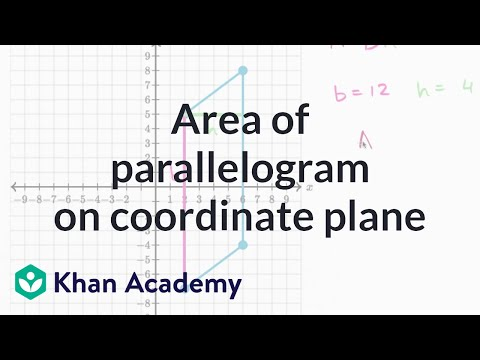Video

# Coordinate plane word problem examples (Full video)

Description: In this word problem, we need to plot the ordered pairs and then figure out the difference in the y coodinate between the two. This will give us our answer! Created by Sal Khan. Plot the two points, and find the distance between Milena's home and the mall. So let's see, she's riding her bicycle from her home at the point negative 3, 4. So let's plot negative 3, 4.

### Other videos you might be interested in### Area of a parallelogram on the coordinate plane (Full video)